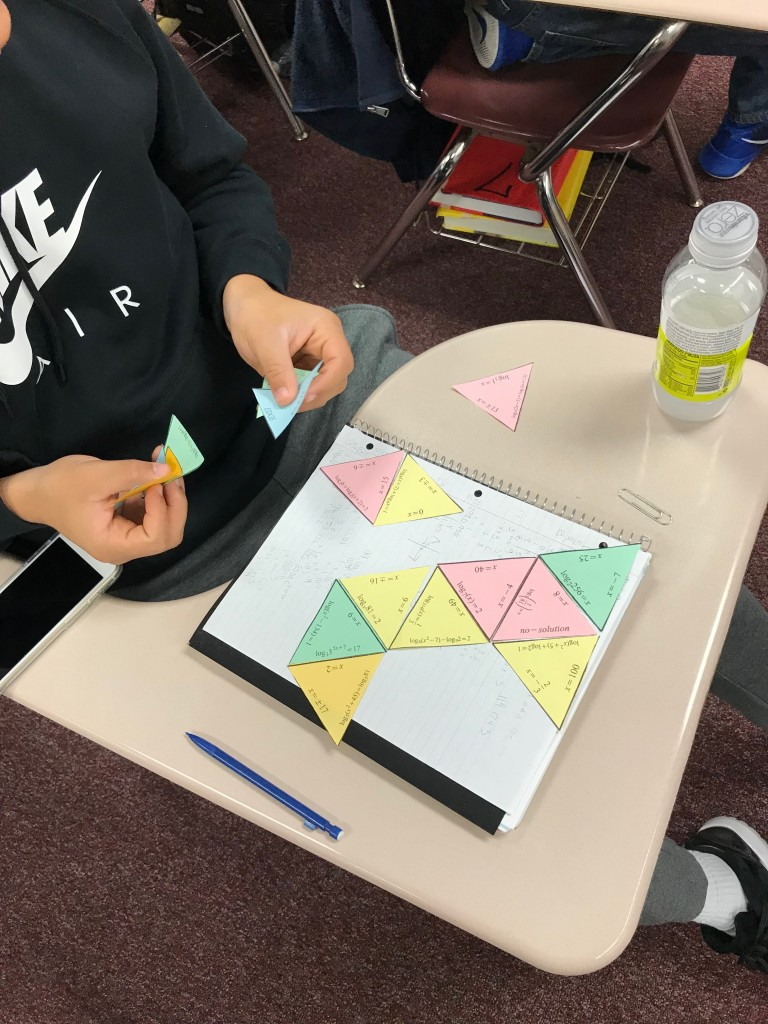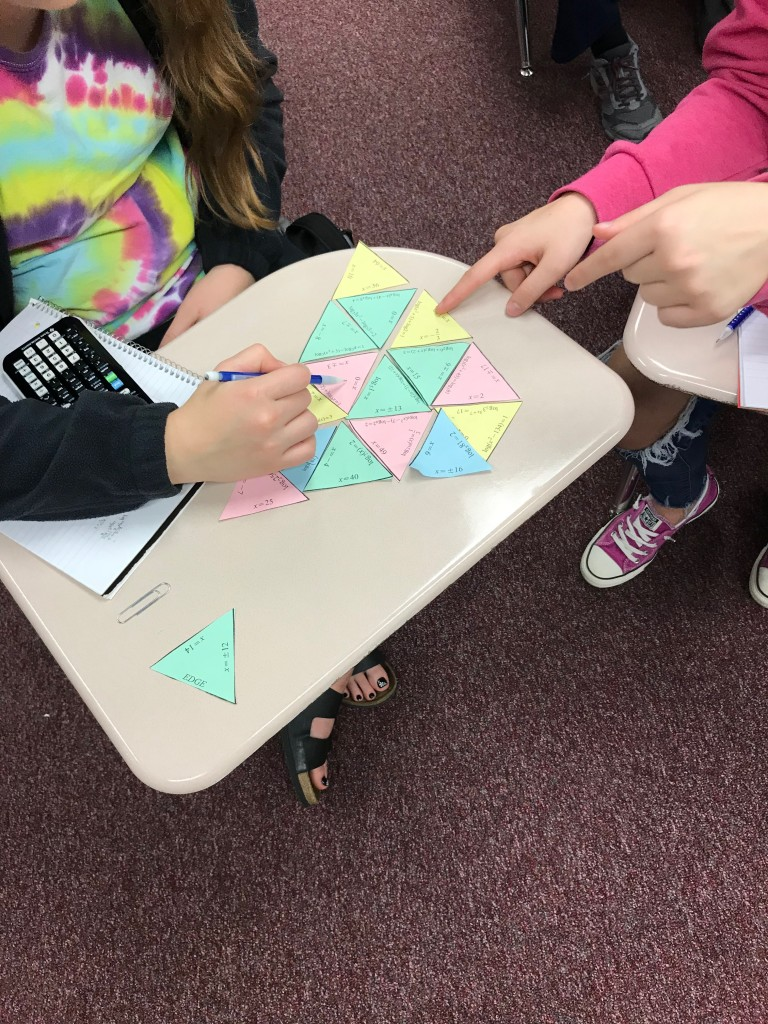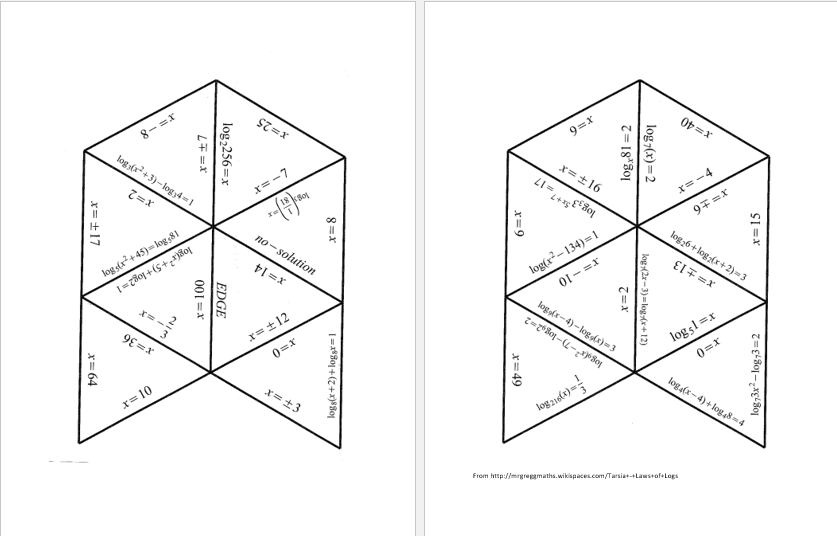# Loving Logarithms

/, Uncategorized/Loving Logarithms

## Loving Logarithms

After solving many, many problems that required finding a and b of an exponential equation of the form y = aebt, we needed to review the basics.

I found a tarsia puzzle on the web. It didn’t include all the types of problems I wanted my precalculus students to review so I found a program to create my own. The idea is that your students cut out the triangles then rearrange them to form an equilateral triangle by matching the answers to the logarithmic equations to the equations.

Feedback from my students has been very positive. They like the challenge. (There are two x = 0 and x = 2 answers.)

Here’s a copy of my puzzle. Here’s the key. I printed the puzzle on multiple colors of paper and then mixed the colors to have some variety. There are 16 triangles that when arranged correctly form one big triangle.By | 2017-11-13T22:01:53+00:00 October 13th, 2017|PreCalculus, Uncategorized|0 Comments

### About the Author: Stacie Bender